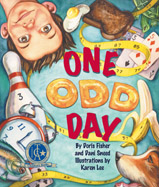Alignment to Standards for AK1 F&R-2. functions, patterns, or sequences by creating patterns involving number, shape, size, rhythm, or color (M4.1.1)
1 N-8. number theory by skip counting by 2's to 20 and 5's and 10's to 100 (M1.1.6)
1 N-9. number theory by identifying odd and even numbers up to 20 (M1.1.6)
2 F&R-1. functions, patterns, or sequences by identifying and continuing patterns, including numbers (M4.1.1)
2 N-7. number theory (skip count by 2's, 5's, or 10's; add or subtract by 10; identify even or odd numbers) (M1.1.6)
3 M1.1.6 Identify, describe, and extend patterns inherent in the number system. Skip count by 2ês, 5ês, and 10ês. Add and subtract by 10. Identify even and odd numbers.
3 M10.1.1 Apply mathematical skills and processes to literature.
3 N-9 number theory by identifying or using patterns in the number system (skip count by 2ês, 5ês, or 10ês; add or subtract by 10; even or odd numbers) (M1.1.6)
5 M4.2.1 Use patterns and their extensions to make predictions and solve problems; describe patterns found in the number system including those formed by multiples, factors, perfect squares, and power of 10.
5 M4.2.4 Use words, lists, and tables to represent and analyze patterns.
K N-12. number theory by demonstrating skip counting by 2's, 5's, and 10's with support (M1.1.6)

Back to Standards Page